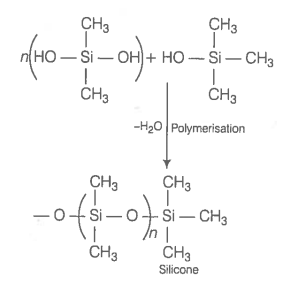Silicon has a strong tendency to form polymers like silicones. The chain length of silicone polymer can be controlled by adding

1.  ${\mathrm{MeSiCl}}_{3}$

2.  ${\mathrm{Me}}_{2}{\mathrm{SiCl}}_{2}$

3.  ${\mathrm{Me}}_{3}\mathrm{SiCl}$

4.  ${\mathrm{Me}}_{4}\mathrm{Si}$

Hint: Trimethylsilyl chloride is used to control the chain length of silicone

Silicon has a strong tendency to form polymers can be controlled by adding ${\mathrm{Me}}_{3}\mathrm{SiCl}$ which block the ends as shown below